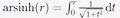Vol.123, No.2, 2020, pp.441-473, doi:10.32604/cmes.2020.08656
OPEN ACCESS
ARTICLE
Addition Formulas of Leaf Functions and Hyperbolic Leaf Functions
• Kazunori Shinohara*
Department of Mechanical Systems Engineering, Daido University, Nagoya, 457-8530, Japan
* Corresponding Author: Kazunori Shinohara. Email: shinohara@06.alumni.u-tokyo.ac.jp
Received 21 September 2019; Accepted 06 February 2020; Issue published 01 May 2020
Abstract
Addition formulas exist in trigonometric functions. Double-angle and half-angle formulas can be derived from these formulas. Moreover, the relation equation between the trigonometric function and the hyperbolic function can be derived using an imaginary number. The inverse hyperbolic functionis similar to the inverse trigonometric function, such as the second degree of a polynomial and the constant term 1, except for the sign − and +. Such an analogy holds not only when the degree of the polynomial is 2, but also for higher degrees. As such, a function exists with respect to the leaf function through the imaginary number i, such that the hyperbolic function exists with respect to the trigonometric function through this imaginary number. In this study, we refer to this function as the hyperbolic leaf function. By making such a definition, the relation equation between the leaf function and the hyperbolic leaf function makes it possible to easily derive various formulas, such as addition formulas of hyperbolic leaf functions based on the addition formulas of leaf functions. Using the addition formulas, we can also derive the double-angle and half-angle formulas. We then verify the consistency of these formulas by constructing graphs and numerical data.
Keywords
Leaf functions; hyperbolic leaf functions; lemniscate functions; Jacobi elliptic functions; ordinary differential equations; nonlinear equations